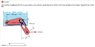# Magnitude of The Force Supported at Point A

• samccain93

## Homework Statement

Calculate the magnitude of the force supported by the point at A under the action of the 2.9-kN load applied to the bracket. Neglect friction in the slot.

ƩMA=0
ƩFy=0
ƩFx=0

## The Attempt at a Solution

I started by finding the moment around A so that I could solve for the vertical force at B:

ΔyAC=(135)sin(34)

MA=0=(2.9)(75.459)-FBy(155)
FBy=-1.41

Then I found FBX using trig functions and the angle between the vertical axis and FB:

(-1.41)tan(56)=-2.09

Then I summed all the forces to find the components of the force at point A:

ƩFy=0=-1.41+FAy
FAy=1.41Kn
ƩFx=0=-2.09+2.99+FAx
FAx=0.9Kn

Then I found FA using the Pythagorean theorem

FA=√(0.92+1.412)=1.672Kn

This was wrong, am I missing a force/forces somewhere, or are my calculations incorrect?

Thanks for any help!

#### Attachments

•Untitled.jpg
18.9 KB · Views: 1,770
Last edited:

## Homework Statement

Calculate the magnitude of the force supported by the point at A under the action of the 2.9-kN load applied to the bracket. Neglect friction in the slot.

ƩMA=0
ƩFy=0
ƩFx=0

## The Attempt at a Solution

I started by finding the moment around A so that I could solve for the vertical force at B:

ΔyAC=(135)sin(34)

MA=0=(2.9)(75.459)-FBy(155)
FBy=-1.41

Then I found FBX using trig functions and the angle between the vertical axis and FB:

(-1.41)tan(56)=-2.09

Then I summed all the forces to find the components of the force at point A:

ƩFy=0=-1.41+FAy →FAy=0.9Kn
check math!
ƩFx=0=-2.09+2.99+FAx →FAx=1.41
0 = -2.09 + 2.9 + FAx
Check typo and math!

•1 person
Good catch Phantom, I fixed it. Doesn't change the final answer though.

## Homework Statement

Calculate the magnitude of the force supported by the point at A under the action of the 2.9-kN load applied to the bracket. Neglect friction in the slot.

ƩMA=0
ƩFy=0
ƩFx=0

## The Attempt at a Solution

I started by finding the moment around A so that I could solve for the vertical force at B:

ΔyAC=(135)sin(34)

MA=0=(2.9)(75.459)-FBy(155)
FBy=-1.41

$\sin \theta = \frac{\mathrm{opposite}}{\mathrm{hypotenuse}}$

$\cos \theta = \frac{\mathrm{adjacent}}{\mathrm{hypotenuse}}$

Given what you're tying to do, are you sure you want to use sin(34) for that?

•1 person
I changed my calculation to 135*sin(34) and re-did all of my calculations to get a final answer of FA=2.09Kn, but that's still wrong.

I changed my calculation to 135*sin(34) and re-did all of my calculations to get a final answer of FA=2.09Kn, but that's still wrong.

What I meant to express is that you are using the sine function. Are you sure that the sine function is the appropriate function here? Maybe a cosine would be better?Gah! Typos are an enemy today. I did use 135*cos(34) and ended up with FA=2.09Kn.

Gah! Typos are an enemy today. I did use 135*cos(34) and ended up with FA=2.09Kn.

Okay, 2.09 kN is the y-component of the force at point A. But there's still the x-component to figure out.

•1 person
The x-component of the force at A = 3.10-2.9 = 0.2

After Pythagorean theorem the force at A comes to 2.099Kn which the system says is incorrect.

I figured it out, I just had to round to 2.10.

Thanks collinsmark!